# CoSMoS R | Complete Stochastic Modelling Solution

#### 2021-05-29

CoSMoS was conceived back in 2009 (see note in section 5) and was officially released on CRAN in April 2019. Since then CoSMoS has become one of the leading and most widely downloaded R packages for stochastic simulation of non-Gaussian time series. Designed with the end user in mind, CoSMoS makes univariate, multivariate, or random field simulations easy. You can reliably generate time series or fields of rainfall, streamflow, wind speed, relative humidity, or any other environmental variable in seconds. Just choose the probability distribution and correlation properties of the time series you want to generate, and it will do the rest.

~Unified Theory of Stochastic Modelling | Papalexiou (2018)

“Hydroclimatic processes come in all “shapes and sizes”. They are characterized by different spatiotemporal correlation structures and probability distributions that can be continuous, mixed-type, discrete or even binary. Simulating such processes by reproducing precisely their marginal distribution and linear correlation structure, including features like intermittency, can greatly improve hydrological analysis and design. … Here, a single framework is proposed that unifies, extends, and improves a general-purpose modelling strategy, based on the assumption that any process can emerge by transforming a specific “parent”" Gaussian process."

~Precise Temporal Disaggregation | Papalexiou et al. (2018)

“Hydroclimatic variables such as precipitation and temperature are often measured or simulated by climate models at coarser spatiotemporal scales than those needed for operational purposes. … Here we introduce a novel disaggregation method, named Disaggregation Preserving Marginals and Correlations (DiPMaC), that is able to disaggregate a coarse-scale time series to any finer scale, while reproducing the probability distribution and the linear correlation structure of the fine-scale process. DiPMaC is also generalized for arbitrary nonstationary scenarios to reproduce time varying marginals.”

~Random Fields Simplified | Papalexiou and Serinaldi (2020)

“Nature manifests itself in space and time. The spatiotemporal complexity of processes such as precipitation, temperature, and wind, does not allow purely deterministic modeling. Spatiotemporal random fields have a long history in modeling such processes, and yet a single unified framework offering the flexibility to simulate processes that may differ profoundly does not exist. Here we introduce a blueprint to efficiently simulate spatiotemporal random fields that preserve any marginal distribution, any valid spatiotemporal correlation structure, and intermittency.”

~Advancing Space-Time Simulation | Papalexiou, Serinaldi and Porcu (2021)

“…we advance random field simulation by introducing the concepts of general velocity fields and general anisotropy transformations. To illustrate the potential of CoSMoS, we simulate random fields with complex patterns and motion mimicking rainfall storms moving across an area, spiraling fields resembling weather cyclones, fields converging to (or diverging from) a point, and colliding air masses.”

# 1 Step by step guide to timeseries simulation

NOTE: For R code chunks running longer than ~10s, we report the CPU time for a Windows 10 Pro x64 laptop with Intel(R) Core(TM) i7-6700HQ CPU @ 2.60GHz, 4-core, 32GB RAM.

In most cases we can accurately simulate a process by reproducing its marginal distribution and its autocorrelation structure. The first is typically represented by a parametric probability distribution; the second either by a parametric correlation structure, or by specific correlation coefficient values. CoSMoS is the first software that enables the generation of time series while preserving any marginal distribution, correlation structure, and intermittency. Intermittency can be defined by the probability of zero values, and is essential in the simulation of precipitation at fine scales (e.g., daily, hourly) but also for any other intermittent process, such as flows of ephemeral streams, wind speed, etc.

Accurate generation of time series requires knowledge of the statistical properties of the process under study. Processes such as precipitation, streamflow, temperature, wind, or evaporation may have very different properties; that is, they can be described by different probability distributions, correlation structures, and may or may not be intermittent.

There are four main steps required to generate a time series: 1. Choosing a probability distribution 2. Choosing a correlation structure, 3. Adding intermittency, and 4. Validating the results.

The four steps are explained below.

## 1.1 Choosing a Probability Distribution

The choice of a probability distribution to describe the variable you wish to simulate is crucial. The probability distribution expresses how frequently specific magnitudes of the variable occur in the data set. Air temperature is typically described by bell-shaped distributions like the Normal. Relative humidity needs distributions defined in the interval (0,1) such as the Beta or the Kumaraswamy. Streamflow typically has heavy tails and power-type distributions might be needed to reproduce the behavior of the extremes. Wind speed typically can be modelled by positively skewed distributions such as the Weibull. Precipitation at fine scales has to be modelled by mixed-type (or else zero inflated) distributions, that is, having a probability value to describe the occurrence of zeros, and a continuous distribution, such the Generalized Gamma or the Burr type XII, to describe the frequency of nonzero values.

CoSMoS supports all the standard distribution functions of R and from other packages (tens of distributions) but also comes with some built-in distributions such as Generalized Gamma, the Pareto type II, and the Burr type III and XII distributions. More details on the CoSMoS distributions and their parameters can be found in Section 4.

Distributions may have differing numbers and types of parameters (e.g. location, scale, and shape parameters). For example, the Generalized Gamma distribution has one scale and two shape parameters.

CoSMoS has built-in functions to fit distributions to data and assess their goodness-of-fit based on graphs; but you can also use any other package for distribution fitting.

## 1.2 Choosing a Correlation Structure

The correlation structure expresses how much the random variables depend upon each other. Processes at fine temporal scales are typically more correlated than those at coarse scales. CoSMoS offers two options to introduce correlations: (1) by defining specific lag autocorrelations as a list starting from lag 0 up to a desired limit, or (2) by using a pre-defined parametric autocorrelation structure.

For example, you can generate a time series with lag-1 autocorrelation $$\rho_1 = 0.8$$ and Generalized Gamma marginal distribution by:

## (i) specifying the sample size
no <- 1000
## (ii) defining the type of marginal distribution and its parameters
marginaldist <- "ggamma"
param <- list(scale = 1, shape1 = .8, shape2 = .8)
## (iii) defining the desired autocorrelation
acf.my <- c(1, 0.8)
## (iv) simulating
ggamma_sim <- generateTS(n = no, margdist = marginaldist, margarg = param, acsvalue = acf.my)
## and (v) visually checking the generated time series
quickTSPlot(ggamma_sim[])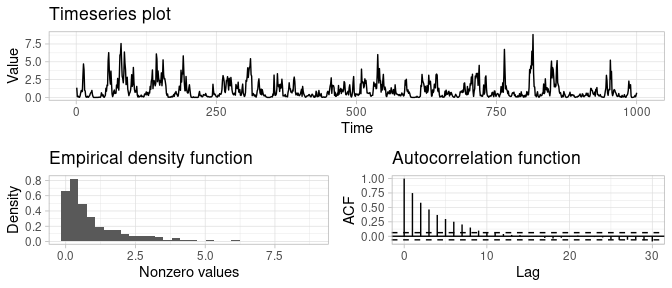You can specify also as many autocorrelation values as you wish. In this example, autocorrelation values are specified up to lag 4:

acf <- c(1, 0.6, 0.5, 0.4, 0.3) #up to lag-4
ggamma_sim <- generateTS(n = no, margdist = marginaldist, margarg = param, acsvalue = acf)
quickTSPlot(ggamma_sim[])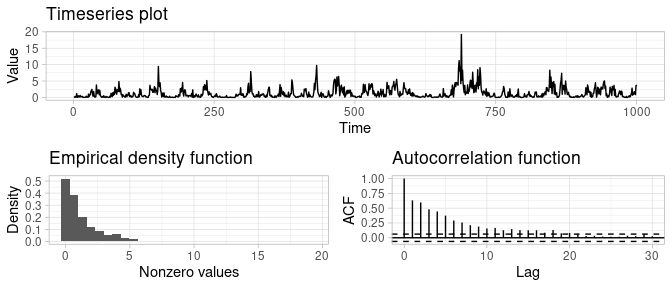A better approach is to use an autocorrelation structure expressed by a parametric function. CoSMoS has built in autocorrelation structures than can be used by the acs() function. You can use the following ACS structures:

• Fractional Gaussian Noise; this is the well-known one-parameter fGn ACS.
• Weibull; a flexible two parameter of exponential form.
• Pareto II: a two parameter power-type ACS.
• Burr XII: a three parameter power-type ACS.

For most practical applications a two-parameter ACS is sufficient; we suggest either the Weibull or the Pareto II.

The acs() function produces autocorrelation values based on any of the four structures and any desired lag. Thus, instead of setting each autocorrelation coefficient explicitly (which might be problematic from a technical viewpoint), we can generate series preserving any one of the four ACSs. For example, you can easily define an ACS and visualize its values up to any lag. Here, all four ACSs are defined and visualized up to lag 10. You can see how changing the ACS function changes how the correlation coefficients decay to zero.

## specify lag
lags <- 0:10

## get the ACS
f <- acs(id = "fgn", t = lags, H = .75)
b <- acs(id = "burrXII", t = lags, scale = 1, shape1 = .6, shape2 = .4)
w <- acs(id = "weibull", t = lags, scale = 2, shape = 0.8)
p <- acs(id = "paretoII", t = lags, scale = 3, shape = 0.3)

## visualize the ACS
dta <- data.table(lags, f, b, w, p)
m.dta <- melt(data = dta, id.vars = "lags")

ggplot(data = m.dta, mapping = aes(x = lags, y = value, group = variable, colour = variable)) +
geom_point(size = 2.5) + geom_line(lwd = 1) +
scale_color_manual(values = c("steelblue4", "red4", "green4", "darkorange"),
labels = c("FGN", "Burr XII", "Weibull", "Pareto II"), name = "") +
labs(x = bquote(lag ~ tau), y = "ACS") + scale_x_continuous(breaks = lags) + theme_light()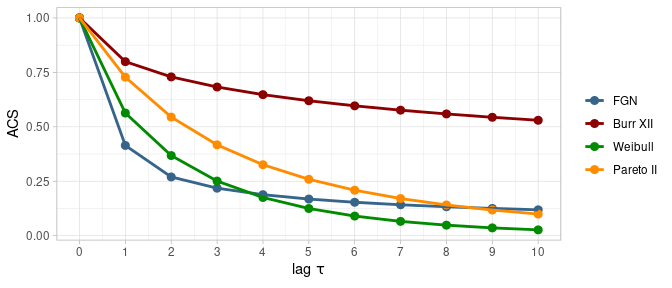We can re-generate the previously generated series which used explicity defined correlations up to lag 4, now with a two parameter Pareto II ACF up to lag 30, which improves the modelling parsimony. More details about the parametric autocorrelation structures can be found in section 3.2 in Papalexiou (2018).

acf <- acs(id = "paretoII", t = 0:30, scale = 1, shape = .75)
ggamma_sim <- generateTS(n = no, margdist = marginaldist, margarg = param, acsvalue = acf)
dta <- data.frame(time = 1:no, value = ggamma_sim[])

quickTSPlot(dta$value)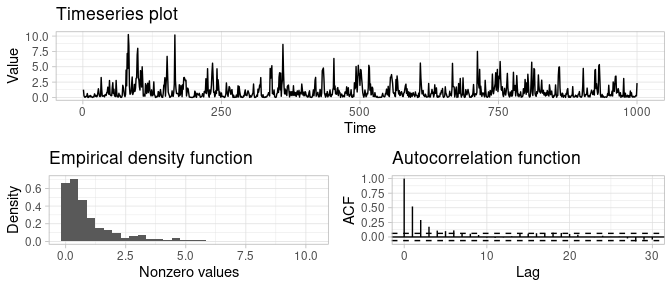Apart from the four autocorrelation functions provided by acs(), we can also create our own. Note that it is important to ensure that the function is positive definite, otherwise the autoregressive model cannot be fitted. This example shows the generation of a time series with the same Generalized Gamma marginal distribution as above, but with a user-defined exponential ACS function up to lag 30. Note that although the time series density is very similar to that in the previous plot, the texture of the time series is very different, due to the changed ACS function. my_acf <- exp(seq(0, -2, -0.1)) ggamma_sim <- generateTS(n = no, margdist = marginaldist, margarg = param, acsvalue = my_acf) quickTSPlot(ggamma_sim[])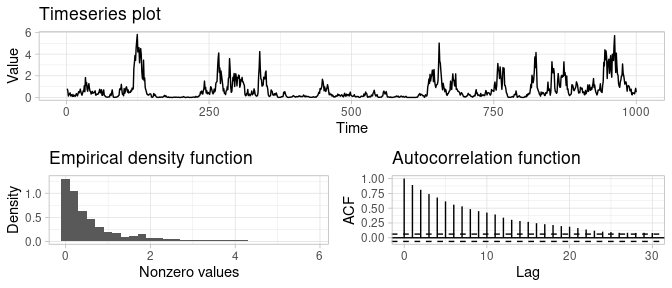## 1.3 Adding Intermittency (if required) CoSMoS easily simulates intermittent processes such as precipitation. The only extra step needed is to define the probability of zero events. For example, say you wish to generate 5 mutually independent timeseries having the previously defined Generalized Gamma distribution with the Pareto II ACS and probability zero p0 = 0.9. The generated data thus will have 90% of zero values (i.e. dry days). prob_zero <- .9 ## the argument TSn = 5 enables the simulation of 5 timeseries. ggamma_sim <- generateTS(n = no, margdist = marginaldist, margarg = param, acsvalue = acf, p0 = prob_zero, TSn = 5) plot(x = ggamma_sim, main = "") + theme_light()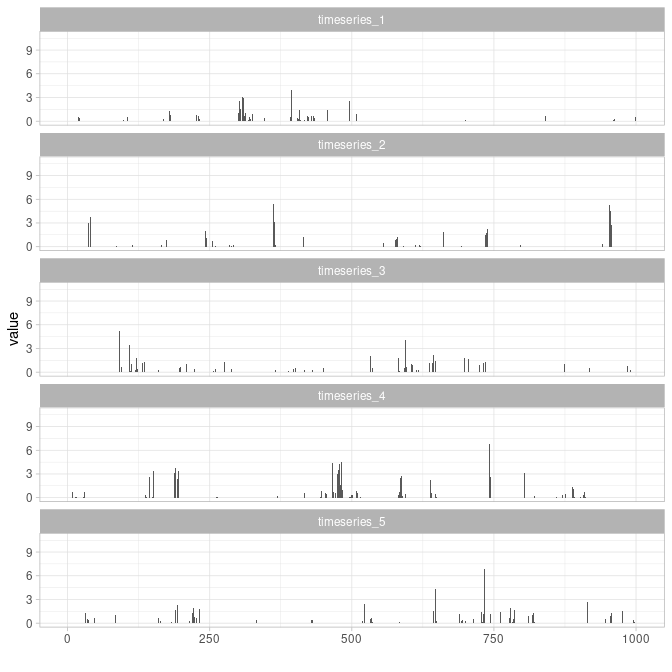## 1.4 Validating the Results You can readily check some basic statistics of the generated time series using the checkTS() function, which return the first three moments, probability zero and the first three autocorrelation coefficients. checkTS(ggamma_sim) ## mean sd skew p0 acf_t1 acf_t2 acf_t3 ## expected 0.11 0.57 8.57 0.90 0.47 0.29 0.21 ## simulation1 0.08 0.40 7.32 0.90 0.42 0.23 0.26 ## simulation2 0.07 0.45 8.44 0.93 0.51 0.45 0.14 ## simulation3 0.10 0.40 6.32 0.88 0.34 0.14 0.12 ## simulation4 0.15 0.69 7.68 0.88 0.50 0.34 0.26 ## simulation5 0.10 0.45 7.45 0.89 0.23 0.04 0.05 ## attr(,"class") ##  "checkTS" "matrix" ## attr(,"margdist") ##  "ggamma" ## attr(,"margarg") ## attr(,"margarg")$scale
##  1
##
## attr(,"margarg")$shape1 ##  0.8 ## ## attr(,"margarg")$shape2
##  0.8
##
## attr(,"p0")
##  0.9

# 2 Multivariate and Random Fields Simulation

The above methods can readily be extended to multidimensional cases, thus enabling the simulation of spatiotemporally correlated random vectors (i.e. correlated timeseries at multiple locations) and random fields (i.e. gridded data), as discussed in detail by Papalexiou and Serinaldi (2020). This requires the definition of suitable spatiotemporal correlation structures (see Porcu et al. (2020) for a thorough review of this topic).

## 2.1 Spatiotemporal Correlation Structures

CoSMoS allows the definition of spatiotemporal correlation functions using the function stcs(), which the spatiotemporal counterpart of the purely temporal acs(). Two classes of spatiotemporal correlation functions are provided:

The stcs() function can produce values of the linear spatiotemporal correlation for any desired time lag and spatial distance using these two correlation function classes, which comprise a variety of structures covering most cases of practical interest. This example shows the Clayton-Weibull spatiotemporal correlation structure:

## specify grid of spatial and temporal lags
d <- 51
st <- expand.grid(0:(d - 1), 0:(d - 1))

## get the STCS
wc <- stcfclayton(t = st[, 1], s = st[, 2], scfid = "weibull", tcfid = "weibull", copulaarg = 2,
scfarg = list(scale = 20, shape = 0.7), tcfarg = list(scale = 5.1,shape = 0.8))

## visualize the STCS
wc.m <- matrix(data = wc, nrow = d)
j <- tail(which(wc.m[1, ] > 0.15), 1)
i <- tail(which(wc.m[, 1] > 0.15), 1)
wc.m <- wc.m[1:i, 1:j]

persp3D(z = wc.m, x = 1: nrow(wc.m), y = 1:ncol(wc.m),
expand = 1, main = "", scale = TRUE, facets = TRUE,
xlab="Time lag", ylab = "Distance", zlab = "STCF", colkey = list(side = 4, length = 0.5),
phi = 20, theta = 120, resfac = 5,  col= gg2.col(100))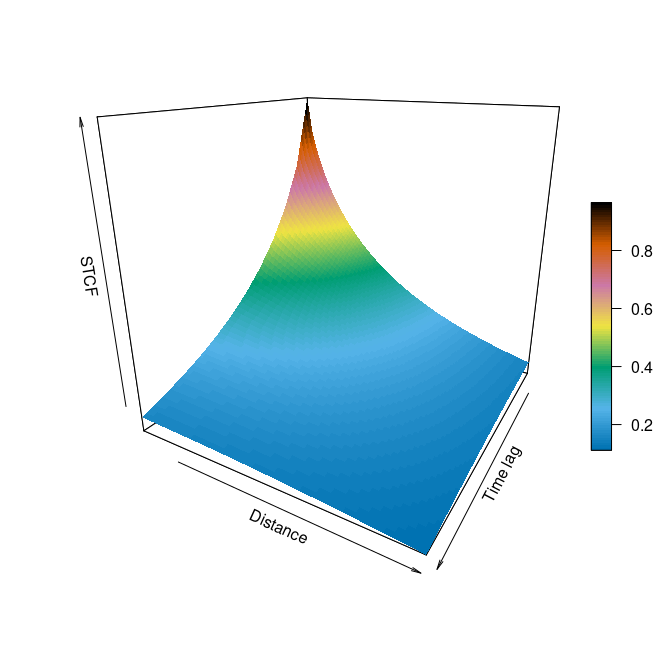## 2.2 Multivariate Time Series Simulation

Similar to the one-dimensional case (i.e. using the function generateTS()), CoSMoS provides the functions generateMTS() and generateMTSFast() to simulate multiple timeseries with specified (identical) marginals and spatiotemporal correlation structures (STCs). Gaussian Vector AutoRegressive (VAR) models are used to reproduce the parent-Gaussian STFC (see Papalexiou (2018) and Papalexiou and Serinaldi (2020)). The VAR parameters see e.g. (Biller and Nelson 2003) corresponding to the desired spatiotemporal correlation function of the target process, are produced by the function fitVAR().

In this example, a set of five random locations is defined that could represent precipitation in five different places. A VAR is then fitted using

• a 4th order process (the spatiotemporal correlations will be preserved up to temporal lag 4),
• the Burr XII marginal distribution,
• a probability zero p0 = 0.8,
• a Clayton-Weibull spatiotemporal correlation structure (see Papalexiou and Serinaldi (2020)), using Weibull functions for both the temporal and spatial correlations.

From the five locations, and the VAR, a set of five timeseries is generated. When the series are plotted, the spatio-temporal correlations among the series can be seen.

## set a sequence of hypothetical coordinates
d <- 5
coord <- cbind(runif(d)*30, runif(d)*30)

## compute VAR model parameters
fit <- fitVAR(spacepoints = coord,
p = 4,
margdist = "burrXII",
margarg = list(scale = 3, shape1 = .9, shape2 = .2),
p0 = 0.8,
stcsid = "clayton",
stcsarg = list(scfid = "weibull", tcfid = "weibull", copulaarg = 2,
scfarg = list(scale = 25, shape = 0.7),
tcfarg = list(scale = 3.1, shape = 0.8) ) )

## generate correlated timeseries
sim <- generateMTS(n = 500, STmodel = fit)

## visualize simulated timeseries
dta <- melt(data = data.table(time = 1:nrow(sim), sim[,1:d]), id.vars = "time")

ggplot(data = dta, mapping = aes(x = time, y = value)) + geom_line() +
facet_grid(facets = variable ~ ., scales = "free_y") + theme_light()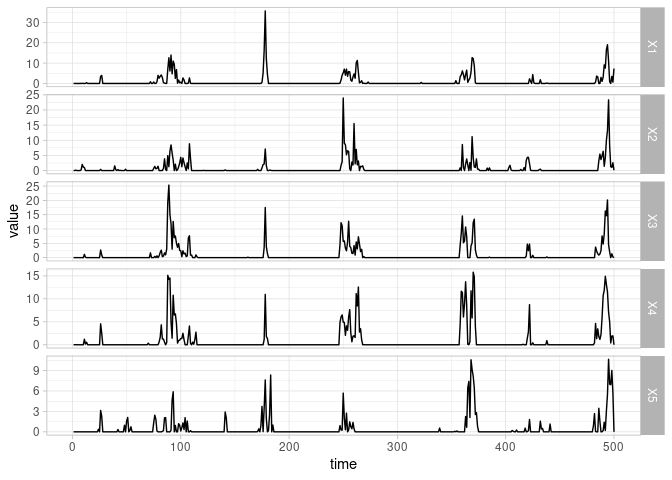The function generateMTSFast() generates multiple time series with approximately separable STCS (Serinaldi and Kilsby 2018), using an algorithm which is computationally less expensive than that in generateMTS(). This allows the simulation of a larger number of cross-correlated and serially dependent timeseries, at the cost of using separable STCSs and accepting some lack of accuracy in the exact reproduction of some terms of the required STCS (Serinaldi and Kilsby 2018).

This example uses generateMTSFast() with similar parameters to the previous example using generateMTS().

## set a sequence of hypothetical coordinates
d <- 5
coord <- cbind(runif(d)*30, runif(d)*30)

## fit and generate correlated timeseries
sim <- generateMTSFast(n = 500,
spacepoints = coord,
p0 = 0.7,
margdist ="burrXII",
margarg = list(scale = 3, shape1 = .9, shape2 = .2),
stcsarg = list(scfid = "weibull", tcfid = "weibull",
scfarg = list(scale = 25, shape = 0.7),
tcfarg = list(scale = 3.1, shape = 0.8)) )

## visualize simulated timeseries
dta <- melt(data = data.table(time = 1:nrow(sim), sim[,1:d]), id.vars = "time")

ggplot(data = dta, mapping = aes(x = time, y = value)) + geom_line() +
facet_grid(facets = variable ~ ., scales = "free_y") + theme_light()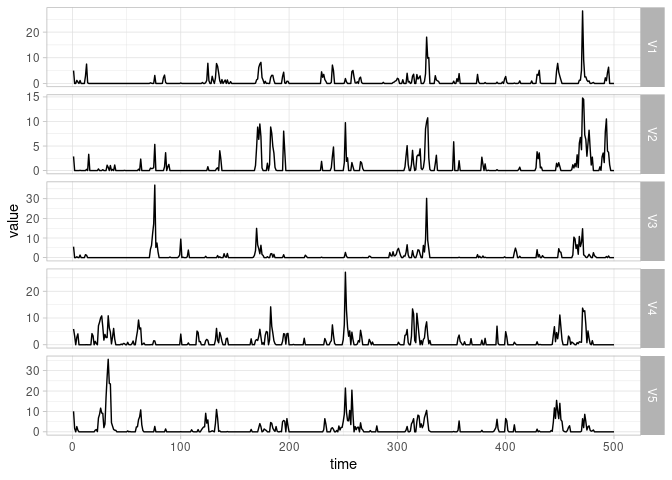## 2.3 Random Fields Simulation

### 2.3.1 Isotropic Random Fields

CoSMoS simulates spatially and temporally correlated isotropic random fields with the functions generateRF() and generateRFFast(), which are analogs of generateMTS() and generateMTSFast(), with the same syntax. The only difference is the specification of the spatial points, which is an integer denoting the side length of a square grid . As was mentioned in the previous section, the algorithm in generateRFFast() is computationally less expensive than that of generateRF(), enabling the simulation of random fields over a greater number of grid points (see Papalexiou (2018), Papalexiou and Serinaldi (2020), and Serinaldi and Kilsby (2018) for more details).

The example below shows the use of both generateRF and generateRFFast. The generation of random fields using generateRF took approximately 16 times as long as using generateRFFast.

## compute VAR model parameters
## CPU time: ~15s
fit <- fitVAR(spacepoints = 20, p = 3, margdist ="burrXII",
margarg = list(scale = 3, shape1 = .9, shape2 = .2), p0 = 0.8, stcsid = "clayton",
stcsarg = list(scfid = "weibull", tcfid = "weibull", copulaarg = 2,
scfarg = list(scale = 20, shape = 0.7), tcfarg = list(scale = 1.1, shape = 0.8) ) )

## generate isotropic random fields with nonseparable correlation structure
sim1 <- generateRF(n = 1000, STmodel = fit)

## fast simulation of isotropic random fields with separable correlation structure
sim2 <- generateRFFast(n = 1000, spacepoints = 20, p0 = 0.7, margdist ="paretoII",
margarg = list(scale = 1, shape = .3),
stcsarg = list(scfid = "weibull", tcfid = "weibull",
scfarg = list(scale = 20, shape = 0.7),
tcfarg = list(scale = 1.1, shape = 0.8)))

### 2.3.2 Validating the Simulated Random Fields

The properties of the generated random fields can be checked with checkRF(). If the parameter method = "stat" (the default), the function returns the first three moments, and the probability zero at 20 grid points. If the parameter method = "statplot", checkRF() returns a multi-panel plot comparing the theoretical and empirical spatial correlation functions at 0, 1, and 2 time lags, the contour plot of the target STCS, and the empirical and target CDFs. If the parameter method = "fields", the function returns level plots of the selected number of simulated random fields (nfields) to visualize the temporal persistence of spatial patterns. If the parameter method = "movie", the fields are written to an animated GIF called “movieRF.gif” in the current working directory.

This example returns the stats, stat plots and fields for the first simulation, which used generateRF.

## check random fields
## CPU time: ~20s
checkRF(RF = sim1, nfields = 9*9, method = "stat")
##                    mean   sd  skew   p0
## expected           0.71 2.69  9.89 0.80
## sample location 1  0.81 2.77  6.50 0.80
## sample location 2  0.88 2.99  6.05 0.79
## sample location 3  0.90 3.64  9.47 0.79
## sample location 4  0.88 3.53 10.27 0.79
## sample location 5  0.82 3.23 10.08 0.80
## sample location 6  0.80 3.02  8.37 0.79
## sample location 7  0.85 3.26  8.08 0.79
## sample location 8  0.90 4.40 16.86 0.80
## sample location 9  0.85 3.34  9.99 0.79
## sample location 10 0.85 3.43  9.70 0.79
## sample location 11 0.82 3.11  7.22 0.80
## sample location 12 0.84 3.32  7.31 0.78
## sample location 13 0.83 3.33  7.98 0.79
## sample location 14 0.86 3.43  7.75 0.80
## sample location 15 0.89 3.72  8.61 0.80
## sample location 16 0.85 3.64  9.52 0.80
## sample location 17 0.83 3.38  8.48 0.79
## sample location 18 0.86 3.71 10.57 0.80
## sample location 19 0.79 3.68 11.20 0.80
## sample location 20 0.84 3.95 11.40 0.80
checkRF(RF = sim1, nfields = 9*9, method = "statplot")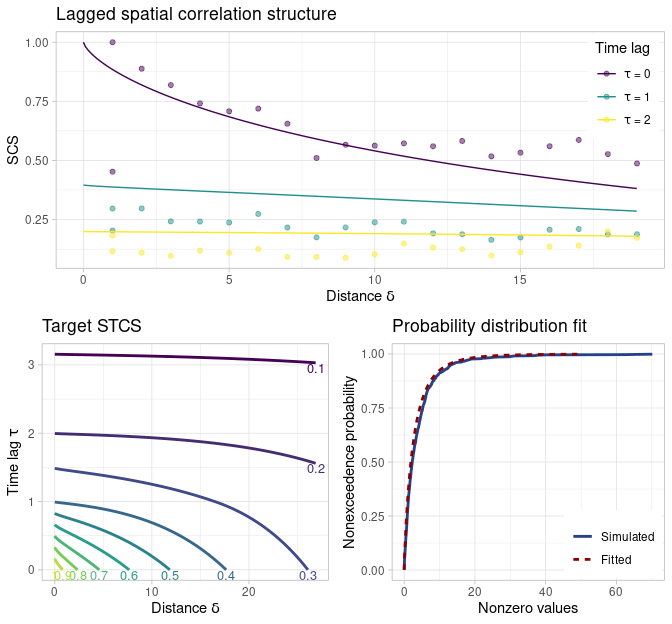checkRF(RF = sim1, nfields = 9*9, method = "field")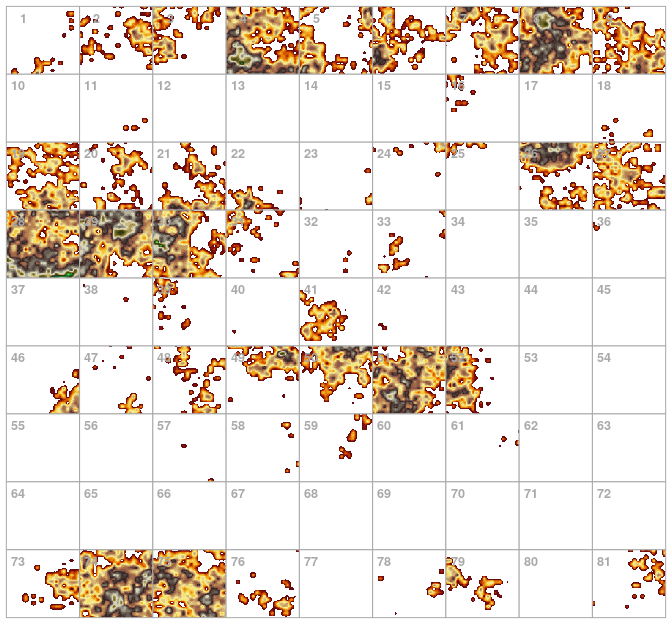### 2.3.3 Anisotropic Random Fields

The functions generateRF() and generateRFFast() allows for the simulation of spatially and temporally correlated anisotropic random fields by specifying the arguments anisotropyid and anisotropyarg when calculating the model parameters via fitVAR(). Three types of anisotropic effects are supported, namely: affine (rotation and stretching), and swirl-like, and “wavy” deformation (see the documentation of the function anisotropyT, Papalexiou, Serinaldi and Porcu (2021), and references therein for more details).

The example below reports the simulation of swirl-like random fields mimicking for instance the shape of atmospheric cyclones. Firstly, one can visualize the the transformed of the coordinate system

## specify a grid of coordinates
m = 30
aux <- seq(0, m - 1, length = m)
coord <- expand.grid(aux, aux)

## transform the coordinate system
at <- anisotropyTswirl(spacepoints = coord, x0 = floor(m / 2), y0 = floor(m / 2),
b = 10, alpha = 1.8 * pi)

## visualize transformed coordinate system
aux = data.frame(lon = at[ ,1], lat = at[ ,2], id1 = rep(1:m, each = m), id2 = rep(1:m, m))
ggplot(aux, aes(x = lon, y = lat)) +
geom_path(aes(group = id1)) + geom_path(aes(group = id2)) + geom_point(col = 2) + theme_light()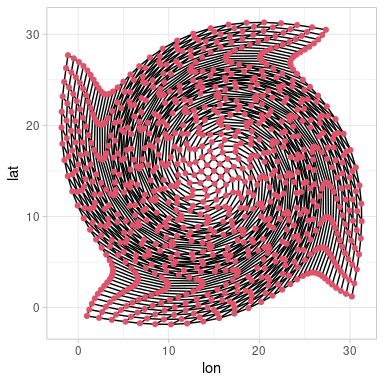Then, one can generate a set of swirl-like random fields with the desired spatiotemporal correlation structure and visualize them

## compute VAR model parameters
fit <- fitVAR(spacepoints = m, p = 1, margdist = 'burrXII',
margarg = list(scale = 3, shape1 = .9, shape2 = .2), p0 = 0.1, stcsid = "clayton",
stcsarg = list(scfid = "weibull", tcfid = "weibull", copulaarg = 2,
scfarg = list(scale = 2, shape = 0.7), tcfarg = list(scale = 20.1, shape = 0.8)),
anisotropyid = "swirl",
anisotropyarg = list(x0 = floor(m / 2), y0 = floor(m / 2), b = 10, alpha = 1.8 * pi) )

## generate
set.seed(9)
sim3 <- generateRF(n=25, STmodel = fit)

## check
checkRF(RF = sim3, nfields = 5*5, method = "field")